Flat morphism

(diff) ← Older revision | Latest revision (diff) | Newer revision → (diff)
A morphism of schemessuch that for any pointthe local ringis flat over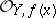(see Flat module). In general, let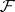be a sheaf of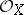-modules; it is called flat overat a pointif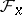is a flat module over the ring. Subject to certain (fairly weak) finiteness conditions, the set of points at which a coherent-moduleis flat overis open in. If, moreover,is an integral scheme, then there exists an open non-empty subset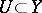such thatis a flat sheaf overat all points lying above.
A flat morphism of finite type corresponds to the intuitive concept of a continuous family of varieties. A flat morphism is open and equi-dimensional (i.e. the dimensions of the fibres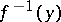are locally constant for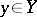). For many geometric properties, the set of pointsat which the fibre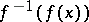of a flat morphismhas this property is open in. If a flat morphismis proper (cf. Proper morphism), then the set of pointsfor which the fibres over them have this property is open .
Flat morphisms are used also in descent theory. A morphism of schemes is called faithfully flat if it is flat and surjective. Then, as a rule, one may check any property of a certain object oversimply by checking this property for the object obtained after a faithfully-flat base change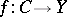. In this connection, interest attaches to flatness criteria for a morphism(or for the-module); herecan be regarded as a local scheme. The simplest criterion relates to the case where the baseis one-dimensional and regular: A coherent-moduleis flat if and only if the uniformizing parameter inhas a trivial annihilator in. In a certain sense the general case is reducible to the one-dimensional case. Letbe a reduced Noetherian scheme and let for any morphism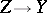, where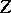is a one-dimensional regular scheme, the base changebe a flat morphism; thenis a flat morphism. Another flatness criterion requires thatis universally open, whileand the geometric fibres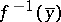are reduced.